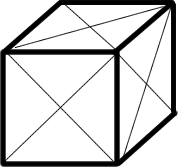#### You may also like### All in the Mind

Imagine you are suspending a cube from one vertex and allowing it to hang freely. What shape does the surface of the water make around the cube?### Just Rolling Round

P is a point on the circumference of a circle radius r which rolls, without slipping, inside a circle of radius 2r. What is the locus of P?### Coke Machine

The coke machine in college takes 50 pence pieces. It also takes a certain foreign coin of traditional design...

# Hamiltonian Cube

### Visiting all the vertices

The figure shows a cube with sides of length $1$, on which all twelve face diagonals have been drawn - creating a network with $14$ vertices (the original eight corners, plus the six face centres) and $36$ edges (the original $12$ edges of the cube plus four extra edges on each face). What is the length of the shortest path along the edges of the network which passes through all $14$ vertices?If you liked this problem, here is an NRICH task which challenges you to use similar mathematical ideas.

This problem is taken from the UKMT Mathematical Challenges.
You can find more short problems, arranged by curriculum topic, in our short problems collection.